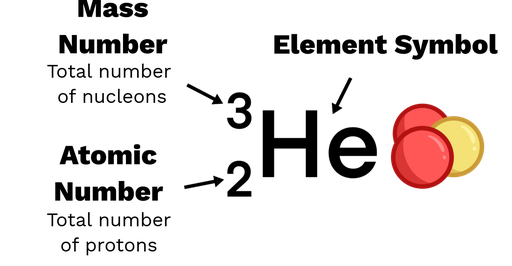The number of protons and neutrons inside a nucleus is represented by nuclide notation (see figure 1 for an example).

• The mass number is the total number of nucleons (protons and neutrons). This is shown in superscript on the left of the element symbol.
• The atomic number is the number of protons, This is shown in subscript on the left of the element symbol.
• We can find the number of neutrons by subtracting the atomic number from the mass number.

The element symbol shows the element's name (for example He is helium). An element will always have the same number of protons, so the atomic number is sometimes omitted. In this case, we may refer to the nuclide 32He as 'helium three'.Figure 1: An annotated helium three nuclide.